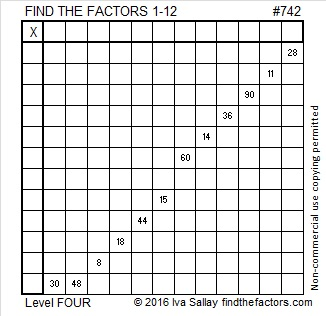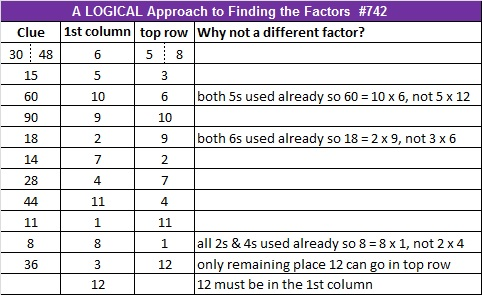# 742 and Level 4

• 742 is a composite number.
• Prime factorization: 742 = 2 x 7 x 53
• The exponents in the prime factorization are 1, 1, and 1. Adding one to each and multiplying we get (1 + 1)(1 + 1)(1 + 1) = 2 x 2 x 2 = 8. Therefore 742 has exactly 8 factors.
• Factors of 742: 1, 2, 7, 14, 53, 106, 371, 742
• Factor pairs: 742 = 1 x 742, 2 x 371, 7 x 106, or 14 x 53
• 742 has no square factors that allow its square root to be simplified. √742 ≈ 27.2396769.Here is a level 4 puzzle for you to try:Print the puzzles or type the solution on this excel file: 12 Factors 2016-01-11

————————————-

Here’s more about composite number 742:

742 can be expressed as the sum of consecutive numbers three ways:

• 184 + 185 + 186 + 187 = 742; that’s 4 consecutive numbers.
• 103 + 104 + 105 + 106 + 107 + 108 + 109 = 742; that’s 7 consecutive numbers.
• 13 + 14 + 15 + 16 + 17 + 18 + 19 + 20 + 21 + 22 + 23 + 24 + 25 + 26 + 27 + 28 + 29 + 30 + 31 + 32 + 33 + 34 + 35 + 36 + 37 + 38 + 39 + 40 = 742; that’s 28 consecutive numbers.

Because 53 is one of its prime factors, 742 is the hypotenuse of Pythagorean triple 392-630-742. The greatest common factor of three numbers in the triple is in the factor pair with 53.

742 is the sum of three squares two different ways:

• 27² + 3² + 2² = 742
• 25² + 9² + 6² = 742

————————————-This site uses Akismet to reduce spam. Learn how your comment data is processed.